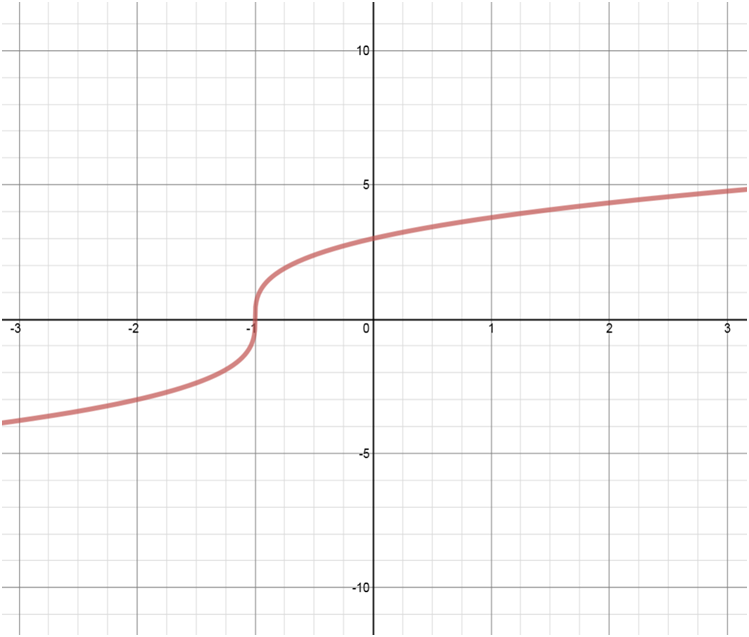# Transformations of functions: Vertical translations

### Transformations of functions: Vertical translations

Vertical translations refer to movements of a graph of a function vertically along the y-axis by changing the y values. So, if y = f(x), then y = (x) + h results in a vertical shift. If h > 0, then the graph shifts h units up; while If h < 0, then the graph shifts h units down.

#### Lessons

• Introduction
An Experiment to Study "Vertical Translations"

Sketch and compare: $\left( y \right) = {x^2}$
VS.
$\left( {y - 3} \right) = {x^2}$
VS.
$\left( {y + 2} \right) = {x^2}$
a)
Sketch all three quadratic functions on the same set of coordinate axes.

b)
Compared to the graph of $y = {x^2}$:
• the graph of $\left( {y - 3} \right) = {x^2}$ is translated "vertically" ________ units _____________.
• the graph of $\left( {y + 2} \right) = {x^2}$ is translated "vertically" ________ units _____________.

• 1.
Vertical Translations
Given the graph of $y=f(x)$ as shown, sketch:
a)
$y = f\left( x \right) - 8$

b)
$y = f\left( x \right) + 3$

c)
In conclusion:
$\left( y \right) \to \left( {y + 8} \right)$: shift ________ units ______________ $\Rightarrow$ all $y$ coordinates _____________________________.
$\left( y \right) \to \left( {y - 3} \right)$: shift ________ units ______________ $\Rightarrow$ all $y$ coordinates _____________________________.